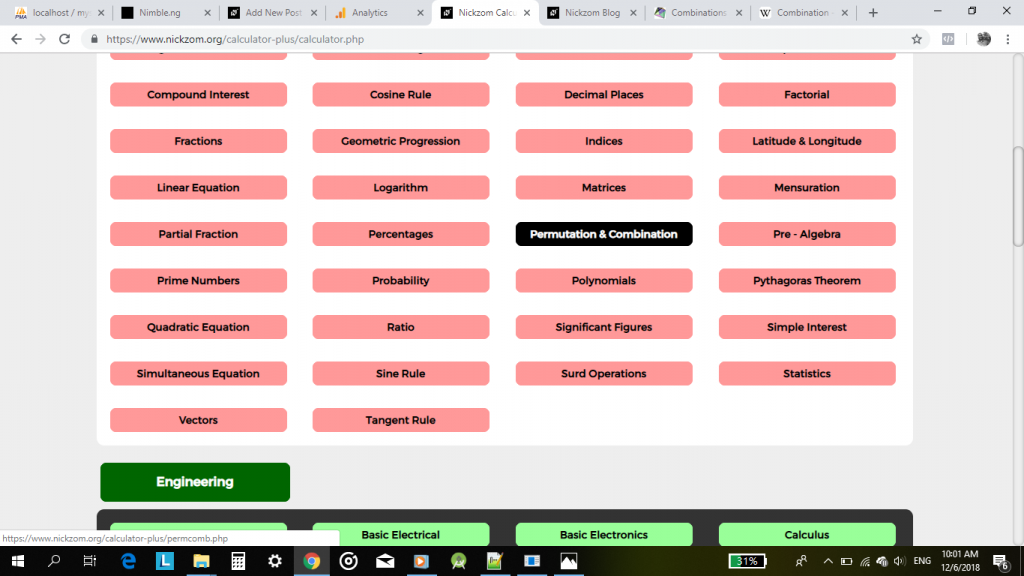## Nickzom Calculator Calculates Permutation and Combination With Steps – The Calculator Encyclopedia

According to Wikipedia,

In mathematics, the notion of permutation relates to the act of arranging all the members of a set into some sequence or order, or if the set is already ordered, rearranging (reordering) its elements, a process called permuting.

In mathematics, a combination is a selection of items from a collection, such that (unlike permutations) the order of selection does not matter.

Getting answers and workings (steps) for permutation and combination problems in Nickzom Calculator – The Calculator Encyclopedia is very simple and I would very much love to show you how you can do this easily.

You can access Nickzom Calculator (The Calculator Encyclopedia) via any of these channels:

First and Foremost, proceed to the Calculator Map, under the Mathematics section click on Permutation|Combination.

Let’s begin with solving a problem on Permutation. I hope by now you do know the value of n and r